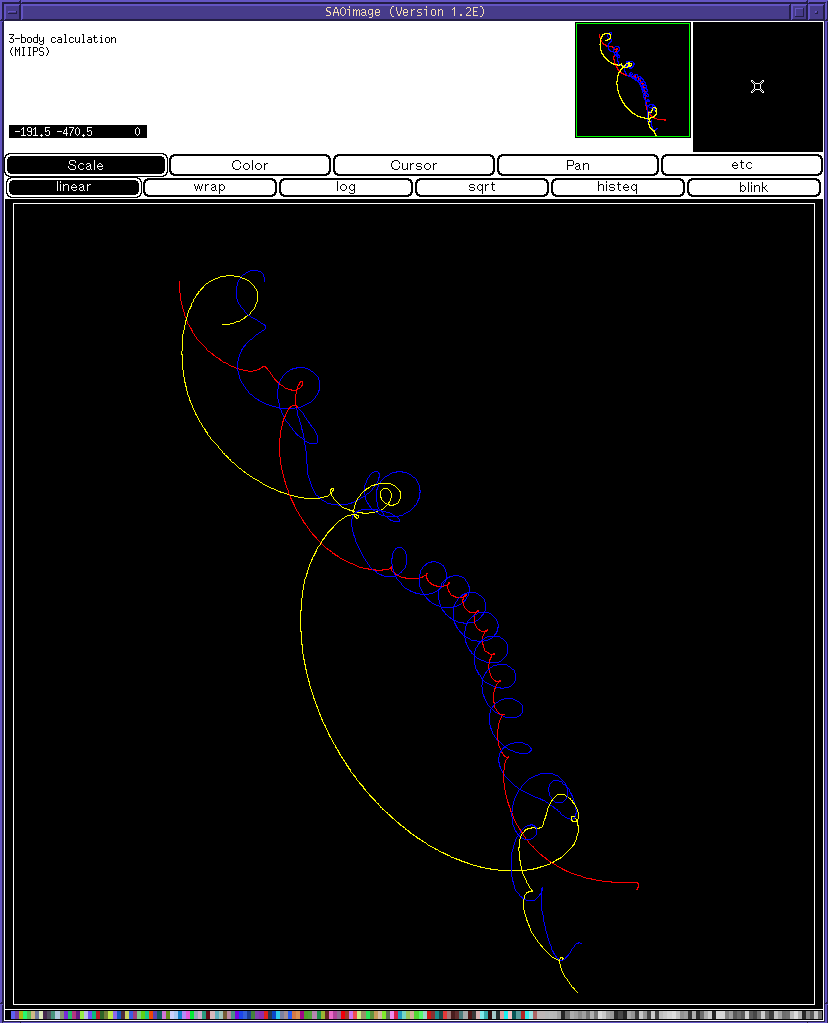## Illustrating Gravitationally Interacting Bodies with "Enhanced" SAOimage

Enhanced SAoimage can be used to illustrate real time gravitaional interactions. In this demo (saodemo8.exe) three masses are assigned starting values of mass, position, and velocity in a plane, and their motion is followed over time.

Here is the dialog produced by the program. In each case, the default value was taken.
``` This program demonstrates 3 gravitationally interacting bodies.  First
run it with the defaults (just press the <CR>).  Then vary the
(delta velocity)/velocity and the minimum time step and notice
how the accuracy of the final loops and slingshots changes.

Be sure that the SAOimage desktop is at least 800 pixels wide

Enter the masses of the 3 objects in solar masses:
(default = 1.0 0.5 0.25)
MASSES=
1.00000
0.500000
0.250000

Enter the initial x and y position of mass 1 in units of AU: (default = 3 0)
POSITION=
3.00000
0.000000E+00

Enter the initial position of mass 2 in units of AU: (default = 0 -3)
POSITION=
0.000000E+00
-3.00000

Enter the initial position of mass 3 in units of AU: (default = -3 0)
POSITION=
-3.00000
0.000000E+00

Enter the initial velocity of object 1 in units of km/sec:  (default = 0 9)
VELOCITY=
0.000000E+00
9.00000

Enter the initial velocity of object 2 in units of km/sec:  (default = 10 0)
VELOCITY=
10.0000
0.000000E+00

Enter the initial velocity of object 3 in units of km/sec:  (default = 0 -10)
VELOCITY=
0.000000E+00
-10.0000

Enter the maximum desired (delta velocity)/velocity
per integration step:  (default = 0.005)
DELTA_VELOCITY/VELOCITY=
0.500000E-02

Enter the minimum allowable time interval in seconds for the calculations:
(default = 2,000;  this takes precedence over the previous constraint)
MINIMUM_TIME_INTERVAL=
2000.00

Enter the number of calculations per plotted point:  (default = 60)
N_STEPS_PER_PLOT=
60

Enter the plotting scale in inches per AU:  (default = 0.15)
PLOT_SCALE=
0.150000

Enter the desired elapsed time in seconds:  (default = 1.5E9)
MAXIMUM_TIME=
0.150000E+10
```